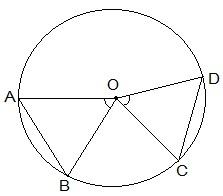Guru

# Recall that two circles are congruent if they have the same radii. Prove that equal chords of congruent circles subtend equal angles at their centres. Q.1

• 1

What is the simplest way for solving the question of class 9th of exercise  10.2 of math of question no.1 give me the best and simple way for solving this question in simple way Recall that two circles are congruent if they have the same radii. Prove that equal chords of congruent circles subtend equal angles at their centres.

Share

1. To recall, a circle is a collection of points whose every point is equidistant from its centre. So, two circles can be congruent only when the distance of every point of both the circles are equal from the centre.For the second part of the question, it is given that AB = CD i.e. two equal chords.

Now, it is to be proven that angle AOB is equal to angle COD.

Proof:

Consider the triangles ΔAOB and ΔCOD,

OA = OC and OB = OD (Since they are the radii of the circle)

AB = CD (As given in the question)

So, by SSS congruency, ΔAOB ΔCOD

∴ By CPCT we have,

AOB = COD. (Hence proved).

• 1